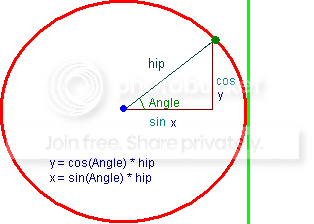## Recommended Posts

If i know x , y, ( blue dot ) angle and length can i find x2 and y2 ( green dot ) for create line ? ( I know that question answer is yes but how ? )Example i know blue dot x : 150 y : 200 and green dot angle 45 and that line length ( hip ) 100.

I'm tried that but not work```#include <GuiConstantsEx.au3>
#include <GDIPlus.au3>

\$hGUI = GUICreate("GDI+", 500, 500)
\$hWnd = WinGetHandle("GDI+")
GUISetState()
_GDIPlus_Startup()
\$hGraphic = _GDIPlus_GraphicsCreateFromHWND(\$hWnd)
\$hPen = _GDIPlus_PenCreate()
Global \$x = 150, \$y = 200
\$Deg = Angle(\$x, \$y, 35, 100)
_GDIPlus_GraphicsDrawLine(\$hGraphic, \$x, \$y, \$Deg, \$Deg, \$hPen)
Do
Until GUIGetMsg() = \$GUI_EVENT_CLOSE
_GDIPlus_PenDispose(\$hPen)
_GDIPlus_GraphicsDispose(\$hGraphic)
_GDIPlus_Shutdown()

Func Angle(\$x1, \$y1, \$Ang, \$Length)
Local \$Return
\$Return = \$x1 + (\$Length * Sin(\$Ang / 180 * 3.14159265358979))
\$Return = \$y1 + (\$Length * Cos(\$Ang / 180 * 3.14159265358979))
Return \$Return
EndFunc   ;==>Angle```

I think my angle function completely wrong but i can't figure out how can i doEdited by Jex

##### Share on other sites

Sorry, I don't have an immediate answer for you, but two things.

First, in your example above don't you want line 12 to be "\$Deg = Angle(\$x, \$y, 45, 100)" ?

Second, for your test run, why not use a 30/60/90 triangle with a hypotenuse of 50 and an angle of either 30 or 60 - then you know the X measurement (either 3 or 4) and the Y measurement (3 or 4)?

##### Share on other sites

http://www.webmath.com/rtri.html

I don't think you can use sin for the X value.

Angle = 60 degrees

Length = 100 px

100 sin 60 = 86.6 <--- Wrong. This is the Y value

Check out this example I put together:

```#include <GUIConstants.au3>

Dim \$GuiSize = 400
Dim \$lineSize = 100
Dim \$GuiCenter = \$GuiSize / 2

GUICreate("My GUI", \$GuiSize, \$GuiSize)  ; will create a dialog box that when displayed is centered

\$sandBox = GUICtrlCreateGraphic (0, 0, \$GuiSize, \$GuiSize)

;Draw centered circle with radius = line length
GUICtrlSetGraphic(-1,\$GUI_GR_COLOR, 0x000000)
GUICtrlSetGraphic(-1,\$GUI_GR_ELLIPSE, \$GuiCenter - \$lineSize, \$GuiCenter - \$lineSize, \$lineSize * 2, \$lineSize * 2)

;Draw line at 0 degrees (from GUI center)
drawLine(0)

;Draw line at 30 degrees (from GUI center)
drawLine(30)

;Draw line at 90 degrees (from GUI center)
drawLine(90)

;Draw line at 180 degrees (from GUI center)
drawLine(180)

GUISetState (@SW_SHOW)

; Run the GUI until the dialog is closed
While 1
\$msg = GUIGetMsg()
If \$msg = \$GUI_EVENT_CLOSE Then ExitLoop
Wend

Func Angle(\$x1, \$y1, \$Ang, \$Length)
Local \$Return
\$Return = \$x1 + (\$Length * Cos(\$Ang / 180 * 3.14159265358979))
\$Return = \$y1 - (\$Length * Sin(\$Ang / 180 * 3.14159265358979))
Return \$Return
EndFunc   ;==>Angle

Func drawLine(\$angle)
\$Deg = Angle(\$GuiCenter, \$GuiCenter, \$angle, \$lineSize)
GUICtrlSetGraphic(-1,\$GUI_GR_MOVE, \$GuiCenter,\$GuiCenter)
GUICtrlSetGraphic(-1,\$GUI_GR_LINE, \$Deg,\$Deg)
EndFunc```
Edited by weaponx

##### Share on other sites

Here is the fixed version of your code. I made a few changes so that the line is drawn from the center of the gui, this makes it easier to verify that it is correct.

```#include <GuiConstantsEx.au3>
#include <GDIPlus.au3>

Dim \$GuiSize = 500
Global \$x = \$GuiSize / 2, \$y = \$GuiSize / 2

\$hGUI = GUICreate("GDI+", \$GuiSize, \$GuiSize)
\$hWnd = WinGetHandle("GDI+")
GUISetState()
_GDIPlus_Startup()
\$hGraphic = _GDIPlus_GraphicsCreateFromHWND(\$hWnd)
\$hPen = _GDIPlus_PenCreate()

;Draw 30 degree angle
\$Deg = Angle(\$x, \$y, 30, 100)
_GDIPlus_GraphicsDrawLine(\$hGraphic, \$x, \$y, \$Deg, \$Deg, \$hPen)

Do
Until GUIGetMsg() = \$GUI_EVENT_CLOSE

_GDIPlus_PenDispose(\$hPen)
_GDIPlus_GraphicsDispose(\$hGraphic)
_GDIPlus_Shutdown()

Func Angle(\$x1, \$y1, \$Ang, \$Length)
Local \$Return
\$Return = \$x1 + (\$Length * Cos(\$Ang / 180 * 3.14159265358979))
\$Return = \$y1 - (\$Length * Sin(\$Ang / 180 * 3.14159265358979))
Return \$Return
EndFunc   ;==>Angle```

##### Share on other sites

Very thanks for your help weaponx.

I'm tried create more simple example :

```#include <GUIConstants.au3>

Dim \$GuiSize = 400, \$LineSize = 150, \$GuiCenter = \$GuiSize / 2

GUICreate("GUI", \$GuiSize, \$GuiSize)
GUICtrlCreateGraphic(0, 0, \$GuiSize, \$GuiSize)
GUICtrlSetGraphic(-1, \$GUI_GR_ELLIPSE, \$GuiCenter - \$LineSize, \$GuiCenter - \$LineSize, \$LineSize * 2, \$LineSize * 2)

For \$i = 1 To 72
DrawLine(\$GuiCenter, \$GuiCenter, \$i * 5, \$LineSize)
Next

GUISetState()

Do
Until GUIGetMsg() = -3

Func DrawLine(\$x1, \$y1, \$Angle, \$Length)
\$x2 = \$x1 + (\$Length * Cos(\$Angle / 180 * 3.14159265358979))
\$y2 = \$y1 - (\$Length * Sin(\$Angle / 180 * 3.14159265358979))
GUICtrlSetGraphic(-1, \$GUI_GR_MOVE, \$x1, \$y1)
GUICtrlSetGraphic(-1, \$GUI_GR_LINE, \$x2, \$y2)
EndFunc   ;==>DrawLine```

## Create an account

Register a new account

• ### Recently Browsing   0 members

×

• Wiki

• Back

• #### Beta

• Git
• FAQ
• Our Picks
×
• Create New...